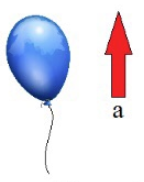# Problem: A balloon filled with helium gas has an average density of ρb = 0.26 kg/m3. The density of the air is about ρa = 1.23 kg/m3. The volume of the balloon is Vb = 0.024 m3. The balloon floats upward with acceleration a. Part (a) Express the net force F on the balloon in terms of its weight W and the buoyancy force Fb on it, assuming upward is the positive direction.Part (b) Calculate the numerical value of the acceleration a in m/s2.

###### FREE Expert Solution

Buoyant force:

$\overline{){{\mathbf{F}}}_{{\mathbf{b}}}{\mathbf{=}}{{\mathbf{\rho }}}_{{\mathbf{a}}}{{\mathbf{V}}}_{{\mathbf{b}}}{\mathbf{g}}}$, where ρa is the density of air, Vb is the volume of the balloon, and g is the acceleration due to gravity.

97% (369 ratings)###### Problem Details

A balloon filled with helium gas has an average density of ρb = 0.26 kg/m3. The density of the air is about ρa = 1.23 kg/m3. The volume of the balloon is Vb = 0.024 m3. The balloon floats upward with acceleration a.Part (a) Express the net force F on the balloon in terms of its weight W and the buoyancy force Fb on it, assuming upward is the positive direction.

Part (b) Calculate the numerical value of the acceleration a in m/s2.